## UGC NET CS 2015 Dec - paper-3

 Question 1
The three outputs x1x2x3 from the 8 X 3 priority encoder are used to provide a vector address of the form 101x1x2x300. What is the second highest priority vector address in hexadecimal if the vector addresses are starting from the one with the highest priority ?
 A BC B A4 C BD D AC
Digital-Logic-Design       Combinational-Circuit
Question 1 Explanation:Question 2
What will be the output at PORT1 if the following program is executed ?
MVI B, 82H
MOV A, B
MOV C, A
MVI D, 37H
OUT PORT1
HLT
 A 37H B 82H C B9H D 00H
Computer-Organization       Machine-Instructions
Question 2 Explanation:
MOV instruction copies the data from one location to other
MVI B, 82H / Copy value 82H to register B
MOV A, B / Copy value of B (82H) to accumulator A
MOV C, A / Copy value of accumulator A (82H) to register C
MVI D, 37H / Copy value 37H to register D
OUT PORT1 /Copy value of accumulator A (82H) to PORT 1 (because still accumulator A is having 82H)
 Question 3
Which of the following 8085 microprocessor hardware interrupt has the lowest priority?
 A RST 6.5 B RST 7.5 C TRAP D INTR
Computer-Organization       Microprocessor
Question 3 Explanation: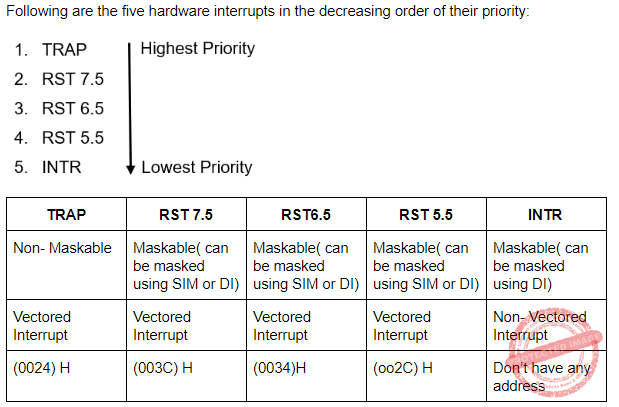DI(Disable Interrupt): DI allows the microprocessor to reject the interrupt. In DI the interrupts are disabled immediately and no flags are affected.
SIM(Set Instruction Mask): It uses flags to disable the interrupts. Here “1” indicated that the interrupt is masked and “0” indicates the the instruction is not masked.
 Question 4
A dynamic RAM has refresh cycle of 32 times per msec. Each refresh operation requires 100 nsec and a memory cycle requires 250 nsec. What percentage of memory’s total operating time is required for refreshes?
 A 0.64 B 0.96 C 2 D 0.32
Digital-Logic-Design       RAM
Question 4 Explanation:Question 5
A DMA controller transfers 32-bit words to memory using cycle stealing. The words are assembled from a device that transmits characters at a rate of 4800 characters per second. The CPU is fetching and executing instructions at an average rate of one million instructions per second. By how much will the CPU be slowed down because of the DMA transfer?
 A 0.6% B 0.12% C 1.2% D 2.5%
Computer-Organization       DMA
Question 5 Explanation:
→ In one second, device is sending - 4800 characters to DMA
→ 1 character = 8 bits
→ So in one second, device is sending - (4800⨯8) bits to DMA
→ In one cycle, DMA sends - 32 bits.
→ To send (4800 ⨯ 8) bits DMA needs = (4800⨯8)/30 cycles = 1200 cycles
→ Now in one second CPU executes 106 instructions.
→ But because of cycle stealing, CPU has slow down and the % by which CPU gets slow down can be calculated as
= 1200/106 * 100
= 12/10000 * 100
=0.12%
 Question 6
A CPU handles interrupt by executing interrupt service subroutine __________.
 A by checking interrupt register after execution of each instruction B by checking interrupt register at the end of the fetch cycle C whenever an interrupt is registered D by checking interrupt register at regular time interval
Computer-Organization       Interrupt-Service-Routing
Question 6 Explanation:
CPU after completing the execution of a instruction checks the status of interrupt if there is a interrupt then CPU executes the interrupt service routine to service it else CPU stats executing next instruction.
 Question 7
Given the following set of prolog clauses :

father(X, Y) :

parent(X, Y),

male(X),

parent(Sally, Bob),

parent(Jim, Bob),

parent(Alice, Jane),

parent(Thomas, Jane),

male(Bob),

male(Jim),

female(Salley),

female(Alice).

How many atoms are matched to the variable ‘X’ before the query father(X, Jane) reports a Result ?

 A 1 B 2 C 3 D 4 E No option is correct.
Artificial-intelligence       Prolog
Question 7 Explanation:
Excluded for evaluation
 Question 8
Forward chaining systems are __________ where as backward chaining systems are __________.
 A Data driven, Data driven B Goal driven, Data driven C Data driven, Goal driven D Goal driven, Goal driven
Artificial-intelligence       backward chaining
Question 8 Explanation:
Forward Chaining: Forward chaining starts with the available data and uses inference rules to extract more data until a conclusion is reached.
It is also known as data driven inference technique.
It is bottom up reasoning.
It is a breadth first search.
For Example: “If it is raining then i will bring the umbrella”. Here “it is raining” is a available data from which more data is extracted and a conclusion “i will bring the umbrella” is drived.
Backward Chaining: Backward chaining is an inference method described colloquially as working backward from the goal.
It is also known as goal driven inference technique.
Here we starts from a goal and apply inference rules to get some data.
It is top down reasoning.
It is a depth first search.
For Example: “If it is raining then i will bring the umbrella”. Here our conclusion is “i will bring the umbrella”. Now If I am bringing an umbrella then it can be stated that it is raining that is why I am bringing the umbrella. So here “ It is raining” is the data obtained from goal . Hence it was derived in a backward direction so it is the process of backward chaining.
 Question 9
Match the following w.r.t. programming language: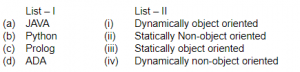A (a)-(iii), (b)-(i), (c)-(ii), (d)-(iv) B (a)-(i), (b)-(iii), (c)-(ii), (d)-(iv) C (a)-(i), (b)-(iii), (c)-(iv), (d)-(ii) D (a)-(ii), (b)-(iv), (c)-(i), (d)-(iii) E None of the above
OOPS       JAVA
Question 9 Explanation:
→ Dynamic programming language is a class of high-level programming languages which, at runtime, execute many common programming behaviors that static programming languages perform during compilation. These behaviors could include extension of the program, by adding new code, by extending objects and definitions, or by modifying the type system.
→ Ada is a structured, statically typed, imperative, and object-oriented high-level computer programming language, extended from Pascal and other languages.
→ Prolog is a logic programming language associated with artificial intelligence and computational linguistics. Prolog is intended primarily as a declarative programming language: the program logic is expressed in terms of relations, represented as facts and rules.
→ Python is dynamically typed and garbage-collected. It supports multiple programming paradigms, including procedural, object-oriented, and functional programming
 Question 10
The combination of an IP address and a port number is known as ___________.
Question 10 Explanation:
Socket address: A socket address is used to uniquely identify a process on a host.
Example: There can be multiple hosts that are communicating to a server. So to identify each host uniquely IP address is required. Now there could be multiple processes executing on a host machine So to identify each process uniquely on a host Port number is used.
So to identify a process on a host combination of an IP address and a port number is used and this combination is termed as Socket Address.
 Question 11
A network with a bandwidth of 10 Mbps can pass only an average of 15,000 frames per minute with each frame carrying an average of 8,000 bits. What is the throughput of this network?
 A 2 Mbps B 60 Mbps C 120 Mbps D 10 Mbps
Computer-Networks       Throughput
Question 11 Explanation:
1 minute - 1500 frames
60 seconds - 1500 frames
1 second - 1500/60 frames
1 second = 25 frames
Now each frame is of 8,000 bits.
So total bits in 1 second = 25 × 80 bits
= 25×8000/1000000 Mbps
= 200/100 Mbps
= 2 Mbps
 Question 12
Consider a subnet with 720 routers. If a three-level hierarchy is chosen with eight clusters, each containing 9 regions of 10 routers, then the total number of entries in the routing table is __________.
 A 25 B 27 C 53 D 72
Computer-Networks       Routing
Question 12 Explanation:
For example, consider a subnet with 720 routers. If there is no hierarchy, each router needs 720 routing table entries.
If the subnet is partitioned into 24 regions of 30 routers each, each router needs 30 local entries plus 23 remote entries for a total of 53 entries.
If a three-level hierarchy is chosen, with eight clusters, each containing 9 regions of 10 routers, each router needs 10 entries for local routers, 8 entries for routing to other regions within its own cluster, and 7 entries for distant clusters, for a total of 25 entries.
 Question 13
In a classful addressing, the IP addresses with 0 (zero) as network number :
 A refers to the current network B refers to broadcast on the local network C refers to broadcast on a distant network D refers to loopback testing E None of the above
Question 13 Explanation:
In a classful addressing, the IP addresses with 0 (zero) as network number refers to the default IP of a host when it has no IP. When a host don’t have any IP then it sends a RARP request by having 0.0.0.0 as source IP.
NOTE: So none of the options is correct but according to UGC official answer keys the correct answer is given as option(A)
 Question 14
In electronic mail, which of the following protocols allows the transfer of multimedia messages?
 A IMAP B SMTP C POP 3 D MIME
Computer-Networks       Application-Layer-Protocol
Question 14 Explanation:
SMTP(Simple Mail Transfer Protocol) is used to send mail containing text, voice, video, to MTA of destination.
MIME(Multimedia Internet Mail Extension) is not a mail protocol. It is used to send mails containing ASCII and Non-ASCII files.
It is an extension of SMTP but not a mail protocol.
POP3(Post Office Protocol) is a client server architecture used to download message from MTA to workstation for offline use.
IMAP(Internet Message Access Protocol) is a client server architecture used to check messages without downloading from MTA.
 Question 15
A device is sending out data at the rate of 2000 bps. How long does it take to send a file of 1,00,000 characters ?
 A 50 B 200 C 400 D 800
Computer-Networks       Bit-rate
Question 15 Explanation:
Explanation: 1 character ------- 8-bits
1,00,000 character ---------- 8,00,000 bits
2000 bits--------- 1 second
1 bit ---------- 1/2000 seconds
8,00,000 bits ---------(1/2000)* 8,00,000
= 400 seconds
 Question 16
In Activity - Selection problem, each activity ai has a start time si and a finish time fi where si ≤ fi. Activities ai and aj are compatible if:
 A si ≥ fj B sj ≥ fi C si ≥ fj or sj ≥ fi D si ≥ fj and sj ≥ fi
Algorithms       Activity-Selection-Problem
Question 16 Explanation:
Each activity ai has a start time si and a finish time fi where 0 ≤ si < fi < ∞. If selected, activity ai takes place during the half-open time interval [ si , fi ]. Activities ai and aj are compatible if the intervals [ si , fi ] and [ sj , fj ] do not overlap. That is, ai and aj are compatible if si ≥ fj or sj ≥ fi. In the activity-selection problem, we wish to select a maximum-size subset of mutually compatible activities.
 Question 17
Given two sequences X and Y :

X = < a, b, c, b, d, a, b >

Y = < b, d, c, a, b, a >

The longest common subsequence of X and Y is :

 A < b, c, a > B < c, a, b > C < b, c, a, a > D < b, c, b, a >
Algorithms       Dynamic-Programming
Question 17 Explanation:Question 18
If there are n integers to sort, each integer has d digits and each digit is in the set {1, 2, ..., k}, radix sort can sort the numbers in :
 A O(d n k) B O(d nk) C O((d +n) k) D O(d (n + k))
Algorithms       Sorting
Question 18 Explanation:
The radix sort running time depends on the stable sort used as the intermediate sorting algorithm. When each digit is in the range 0 to k-1(It takes k possible values), and ‘k’ is not too large, counting sort is the obvious choice. Each pass over n d-digits numbers then takes time O(n+k). There are ‘d’ passes, and so total time for radix sort is O(d (n + k))
 Question 19
The solution of the recurrence relationA O(lg n) B O(n) C O(n lg n) D None of the above
Algorithms       Asymptotic-Complexity
Question 19 Explanation:
Assume T(n) = O(1) for small n≤80.
T(n) ≤ T(n/5)+T((7n/10)+6)+O(n)
Inductively verify that T(n)≤cn for some constant c.
T(n) ≤ c(n/5)+c(7n/10+6)+O(n)
≤ 9cn/10+6c+O(n)
≤ cn
In above, choose c so that c((n/10)−6) beats the function O(n) for all n.
Note: They given ‘s’ instead 5.
 Question 20
Floyd-Warshall algorithm utilizes __________ to solve the all-pairs shortest paths problem on a directed graph in __________ time.
 A Greedy algorithm, θ (V3) B Greedy algorithm, θ (V2 lgn) C Dynamic programming, θ (V3) D Dynamic programming, θ (V2 lgn)
Algorithms       Sorting
Question 20 Explanation:
Floyd-Warshall algorithm utilizes Dynamic Programming to solve the all-pairs shortest paths problem on a directed graph in θ(V3) time, where “V” is the number of vertices.
 Question 21
Let n = 4 and (a1, a2, a3, a4) = (do, if, int, while). Let p(1 : 4) = (3/8, 3/8, 1/8, 1/8) and Let q(0 : 4) = (2/8, 3/8, 1/8, 1/8, 1/8) where p(i) and q(i) denotes the probability with which we search ai and the identifier x being searched satisfy ai < x < ai+1 respectively. The optimal search tree is given by:
 ABCD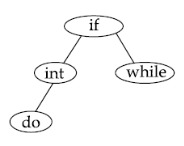Engineering-Mathematics       Probability
Question 21 Explanation:
The above problem is nothing but Optimal Binary Search Tree(OBST). The OBST we are using the recurrence relation is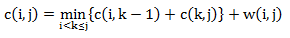Initially, we have w(i,j)=q(i), c(i,j)=0 and r(i,i)=0, 0 ≤ i ≤ 4. The observations are w(i,j)=p(j)+q(j)+w(i,j-1), we get
w(0,1) = p(1) + q(1) + w(0,0) = 8
c(0,1) = w(0,1) + min{c(0,0) + c(1,1)} = 8
r(0,1) = 1
w(1,2) = p(2) + q(2) + w(1,1) = 7
c(1,2) = w(1,2) + min{c(1,1) + c(2,2)} = 7
r(0,2) = 2
w(2,3) = p(3) + q(3) + w(2,2) = 3
c(2,3) = w(2,3) + min{c(2,2) + c(3,3)} = 3
r(2,3) = 3
w(3,4) = p(4) + q(4) + w(3,3) = 3
c(3,4) = w(3,4) + min{c(3,3) + c(4,4)} = 3
r(3,4) = 4 knowing w(i, i+1) and c(i, i+1), 0 ≤ i < 4 and to compute recurrence relation of OBST and compute w(i, i+2), c(i,i+2) and r(i,i+2), 0 ≤ i < 3. This process can repeated until w(0,4), c(0,4) and r(0,4) are obtained.Question 22
The family of context sensitive languages is __________ under union and __________ under reversal.
 A closed, not closed B not closed, not closed C closed, closed D not closed, closed
Theory-of-Computation       Languages-and-Grammars
Question 22 Explanation:
Context sensitive languages are closed under union, intersection, complement, concatenation, kleene star, ϵ-free Homomorphism, substitution( ϵ-free), inverse homomorphism, reverse, intersection with regular language.
Hence the correct option is (C)
 Question 23
Match the following:A (a)-(i), (b)-(ii), (c)-(iii), (d)-(iv) B (a)-(i), (b)-(ii), (c)-(iv), (d)-(iii) C (a)-(iv), (b)-(iii), (c)-(ii), (d)-(i) D (a)-(iv), (b)-(iii), (c)-(i), (d)-(ii)
Theory-of-Computation       Languages-and-Grammars
Question 23 Explanation:
{an bn|n > 0} is a deterministic context free language but not regular because the given language requires a storing device and finite state machine does not contain any storing device. {an bn|n > 0} can be accepted by a pushdown automata by pushing all the a’s on top of stack and when b’s come as input pop one “a” for each “b”.
The given language {an bn an. | n > 0} is a context sensitive language because for this language if we push a’s on top of stack and for every “b” we will pop a single “a” from top of stack and when after “b” again “a” will come as input then that time stack will be empty and we can’t compare number of a’s that come after “”b”. But we can have a linear bounded automata that can accept the given language by moving the read/write head accordingly.
Since the given language is CSL and CSL are closed under complement so given language can not be accepted by a deterministic pushdown automation {an bn an} is context sensitive language but not context free language, the logic is the same as above. SInce for given language can’t be accepted by pushdown automata, so it is not a context free language.
 Question 24
The language of all non-null strings of a’s can be defined by a context free grammar as follows: S → aS|Sa|a The word a3 can be generated by __________ different trees.
 A two B three C four D five
Theory-of-Computation       Context-Free-Grammar
Question 24 Explanation:Question 25
Which one of the following non-functional quality attributes is not highly affected by the architecture of the software?
 A Performance B Reliability C Usability D Portability
Software-Engineering       Software-quality
Question 25 Explanation:
Performance: Performance refers to the response of the system while performing a certain actions in certain period of time. A software can perform better only if it have well structured,easy to maintain architecture.
Reliability: Reliability refers to the ability of a software to continue to operate in the presence of a predefined condition. A software can be reliable if it’s architecture is good enough to work well in inappropriate situations.
Usability: Usability refers to the ease in using the software. The usability is not related to the architecture of the software no matter how complex or simple the software architecture is but the main thing is should be easy to use by the user.
Portability: It refers to the ability to port the software to different platforms or systems. The architecture of a software should be in a way that it can be used at different platforms.
Other than usability all given attributes are somewhere affected by the architecture of the software.
 Question 26
The context free grammar given by
S → XYX
X → aX|bX|λ
Y → bbb
generates the language which is defined by regular expression:
 A (a + b)*bbb B abbb(a + b)* C (a + b)*(bbb)(a + b)* D (a + b)(bbb)(a + b)*
Theory-of-Computation       Context-Free-Grammar
Question 26 Explanation:
Option(a) is not correct because S → XYX, Y → bbb it means the regular expression for given grammar will contain "bbb" in between.
Option(B) is incorrect because "bbbb(a+b)* can also be generated by given grammar.
Option (C) is correct because S → XYX X → aX|bX|λ Y → bbb , here "bbb" will be in middle and X → aX|bX|λ can generate (a+b)* and since S → XYX is having "X" before and after "Y" so it is correct.
Option (D) is not correct because X → aX|bX|λ can generate (a+b)^* and since S → XYX is having "X" before and after "Y" so we can have (a+b)* before and after "bbb"
 Question 27
There are exactly __________ different finite automata with three states x, y and z over the alphabet {a, b} where x is always the start state.
 A 64 B 56 C 1024 D 5832
Theory-of-Computation       Finite-Automata
Question 27 Explanation: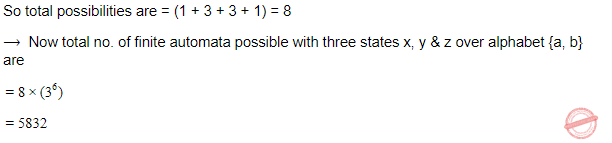Question 28
Given the following two languages: L1 = {an b an |n > 0} L2 = {an b an bn + 1|n > 0} Which of the following is correct ?
 A L1 is context free language and L2 is not context free language B L1 is not context free language and L2 is context free language C Both L1 and L2 are context free languages D Both L1 and L2 are not context free languages
Theory-of-Computation       Context-Free-Language
Question 28 Explanation:
L1 is CFL because we can push all a's first on the top of stack when a "b" come as input change the state and don't push "b" on stack now when "a" comes after "b" then for each "a" pop one "a" from top of stack.
L2 is not CFL because we can push all a's first on the top of stack when a "b" come as input change the state and don't push "b" on stack now when "a" comes after "b" then for each "a" pop one "a" from top of stack. . Bur for "b" that comes in last we don't have "a" to compare them. So it is not CFL language.
 Question 29
Which of the following is used to make an Abstract class?
 A Making at least one member function as pure virtual functions B Making at least one member function as virtual function C Declaring as Abstract class using virtual keyword D Declaring as Abstract class using static keyword
OOPS       Properties
Question 29 Explanation:
Abstract class may consist of at least one abstract method.
Abstract method means method without body, we can also be called as pure virtual functions.
 Question 30
Match the following with reference to object oriented modelling:A (a)-(iv), (b)-(iii), (c)-(i), (d)-(ii) B (a)-(iii), (b)-(iv), (c)-(i), (d)-(ii) C (a)-(iii), (b)-(i), (c)-(ii), (d)-(iv) D (a)-(iv), (b)-(iii), (c)-(ii), (d)-(i)
OOPS       Properties
Question 30 Explanation:
Polymorphism: Polymorphism means many forms. It is used to perform similar operations to do similar things.
Inheritance: It is the process of creating new classes(derived class) from existing classes( base class) by inheriting their properties into derived class.
Encapsulation:It is the process of Picking both operator and attributes with operations appropriate to model an object
Abstraction: Abstraction refers to the process of Hiding implementation details of methods from users of objects
 Question 31
In CRC based design, a CRC Team consists of:
(a)one or two users representatives
(b)several programmers
(c)project coordinators
(d)one or two system analysts
 A (a) and (c) B (a), (b), (c) and (d) C (a), (c) and (d) D (a), (b) and (d)
Computer-Networks       Error-Control-Methods
Question 31 Explanation:
Critical review committee(CRC) should have:
(a)one or two users representatives
(b)project coordinators
(c)one or two system analysts
In a CRC team there is no need of a programmer. Hence Option(C) is the correct answer.
 Question 32
The end points of a given line are (0, 0) and (6, 18). Compute each value of y as x steps from 0 to 3, by using equation of straight line:
 A For x = 0, y = 0; x = 1, y = 3; x = 2, y = 6; x = 3, y = 9 B For x = 0, y = 1; x = 1, y = 3; x = 2, y = 4; x = 3, y = 9 C For x = 0, y = 2; x = 1, y = 3; x = 2, y = 6; x = 3, y = 9 D For x = 0, y = 0; x = 1, y = 3; x = 2, y = 4; x = 3, y = 6
Engineering-Mathematics       Co-ordinate-Geometry
Question 32 Explanation:Question 33
Which of the following graphic primitives are considered as the basic building blocks of computer graphics ?
(a)Points
(b)Lines
(c)Polylines
(d)Polygons
 A (a) only B (a) and (b) C (a), (b) and (c) D (a), (b), (c) and (d)
Computer-Graphics       Basic-Building-Blocks
Question 33 Explanation:
Ponits, lines and polygon lines are the basic building blocks of computer graphics
 Question 34
Javascript and Java has similar name because __________ is/are true.

(a)Javascripts syntax is loosely based on Java’s syntax

(b)Javascript is stripped down version of Java

(c)Java and Javascript are originated from the Island of Java

 A (a) only B (a), (b) and (c) C (a) and (b) D (a) and (c)
Web-Technologies       Java-Script
Question 34 Explanation:
→ Java programming language whereas Javascript is script language.
→ Java can used to create the application independently.
→ JavaScript is used along with HTML mainly meant for validation.
 Question 35
Which of the following statements are true with reference to the way of describing XML data?
(a)XML uses DTD to describe the data
(b)XML uses XSL to describe the data
(c)XML uses a description node to describe the data
 A (a) only B (b) only C (a) and (b) D (a) and (c)
Web-Technologies       XML
Question 35 Explanation:
Option(A): XML uses DTD to define the structure of an XML document. It defines the structure with a list of legal elements. Hence statement (a) is correct.
Option(B): XSLT (eXtensible Stylesheet Language Transformations) is the recommended style sheet language for XML.XSLT is far more sophisticated than CSS. With XSLT you can add/remove elements and attributes to or from the output file. You can also rearrange and sort elements, perform tests and make decisions about which elements to hide and display, and a lot more. XSLT uses XPath to find information in an XML document.
Hence here XML is not using XSL to describe the data. So statement (b) is wrong.
Option(C): Everything in an XML document is a node:
The entire document is a document node
Every XML element is an element node
The text in the XML elements are text nodes
Every attribute is an attribute node
Hence statement (c) is correct.
 Question 36
Which of the following is/are correct with reference to Abstract class and interface ?
(a)A class can inherit only one Abstract class but may inherit several interfaces.
(b)An Abstract class can provide complete and default code but an interface has no code.
 A (a) is true B (b) is true C Both (a) and (b) are true D Neither (a) nor (b) is true
OOPS       Properties
Question 36 Explanation:
Statement (a) is true.
An abstract class may or may not have abstract methods so that it may provide the complete and default code.
 Question 37
Match the following with respect to various memory management algorithms:A (a)-(iii), (b)-(iv), (c)-(ii), (d)-(i) B (a)-(ii), (b)-(iii), (c)-(i), (d)-(iv) C (a)-(iv), (b)-(iii), (c)-(ii), (d)-(i) D (a)-(ii), (b)-(iii), (c)-(iv), (d)-(i)
Operating-Systems       Memory-Management
Question 37 Explanation:
Working set is used to provide frames according to the dynamically changing requirements of a process in the main memory. Here pages are stored in the main memory on demand i.e.only when a page is required for execution of a process it is allocated a frame into main memory.
Segmentation supports the user view of memory because it divides a process into segments in such a way that the meaning of the code does not change after dividing it.
Dynamic partitioning helps in eliminating the internal fragmentation by providing the partitions dynamically but using this method of partitioning external fragmentation can’t be avoided. So compaction is a method used to overcome the external fragmentation problem in dynamic partitioning.
Using fixed partitioning we can store a process in fixed partitions of main memory. By doing this only the pages required for the successful execution of a process are bring into memory and this way instead of storing only a single process in main memory, multiple processes can be stored now. Hence degree of multiprogramming increases.
 Question 38
Function of memory management unit is:
 A Address translation B Memory allocation C Cache management D All of the above
Operating-Systems       Memory-Management
Question 38 Explanation:
Memory management unit is used for converting the logical address into the physical address. It does not allocate memory or it is not responsible for the cache management. It’s task is to compare the logical address with the limit if it is less than the limit, it adds the logical address to the base value and provides the physical address.Question 39
Consider a system with twelve magnetic tape drives and three processes P1, P2 and P3. Process P1 requires maximum ten tape drives, process P2 may need as many as four tape drives and P3may need up to nine tape drives. Suppose that at time t1, process P1 is holding five tape drives, process P2is holding two tape drives and process P3 is holding three tape drives. At time t1, system is in:
 A safe state B unsafe state C deadlocked state D starvation state
Question 39 Explanation:Question 40
In Unix operating system, special files are used to:
 A buffer data received in its input from where a process reads B provide a mechanism to map physical device to file names C store list of file names plus pointers associated with i-nodes D store information entered by a user application program or utility program
Operating-Systems       UNIX-Operating-System
Question 40 Explanation:
Special files: Special files in UNIX operating system are used for I/O devices. In the file system they appear like an ordinary file or a directory. Special files are used to store information about the I/O devices.
 Question 41
Match the following in Unix file system:A (a)-(iv), (b)-(i), (c)-(ii), (d)-(iii) B (a)-(i), (b)-(iii), (c)-(ii), (d)-(iv) C (a)-(iii), (b)-(i), (c)-(ii), (d)-(iv) D (a)-(iv), (b)-(ii), (c)-(i), (d)-(iii)
Operating-Systems       UNIX-Operating-System
Question 41 Explanation:
Boot block contains the code for making OS ready.
Super block contains information about file.
Inode contains information about file system.
Data block contains info regarding data storage.
 Question 42
In an operating system, indivisibility of operation means:
 A Operation is interruptible B Race - condition may occur C Processor can not be preempted D All of the above
Operating-Systems       Process-Synchronization
Question 42 Explanation:
Indivisibility of operation refers to the irreducibility of an operation i.e either an operation will be executed completely or it will not be executed.
So we can say that indivisibility of operation means Processor can not be preempted because a non-pre-empted operation is the one which is either not executed but if its execution starts then it can’t be interrupted until it executes completely.
 Question 43
A horn clause is __________.
 A A clause in which no variables occur in the expression B A clause that has at least one negative literal C A disjunction of a number of literals D A clause that has at most one positive literal
Engineering-Mathematics       Horn-Clause
Question 43 Explanation:
A Horn clause is a clause(a disjunction of literals) with at most one positive literal.
Any Horn clause therefore belongs to one of four categories:
A rule: 1 positive literal, at least 1 negative literal.
A fact or unit: 1 positive literal, 0 negative literals.
A negated goal : 0 positive literals, at least 1 negative literal.
The null clause: 0 positive and 0 negative literals.
 Question 44
In Propositional Logic, given P and P → Q, we can infer __________.
 A ~ Q B Q C P ∧ Q D ~ P ∧ Q
Engineering-Mathematics       Propositional-Logic
Question 44 Explanation:
Modus ponens rule / Rule of detachment: According to Modus ponens rule given P and P → Q, we can infer Q
 Question 45
Reasoning strategies used in expert systems include __________.
 A Forward chaining, backward chaining and problem reduction B Forward chaining, backward chaining and boundary mutation C Forward chaining, backward chaining and back propagation D Backward chaining, problem reduction and boundary mutation
Artificial-intelligence       Expert-Systems
Question 45 Explanation:
Expert systems: are designed to solve complex problems by reasoning through bodies of knowledge, represented mainly as if–then rules rather than through conventional procedural code.
So we can say that expert systems are used for problem reduction.
For problem reduction an expert system can use forward or backward chaining.
Forward Chaining: Forward chaining starts with the available data and uses inference rules to extract more data until a conclusion is reached.
It is also known as data driven inference technique.
It is bottom up reasoning.
It is a breadth first search.
Backward Chaining: Backward chaining is an inference method described colloquially as working backward from the goal.
It is also known as goal driven inference technique.
Here we starts from a goal and apply inference rules to get some data.
It is top down reasoning.
It is a depth first search.
 Question 46
Language model used in LISP is __________.
 A Functional programming B Logic programming C Object oriented programming D All of the above
Artificial-intelligence       LISP
Question 46 Explanation:
LISP is functional language. A functional language:
Uses declarative programming model.
Focus is on “ what you are doing”
Supports parallel programming.
It’s functions have no side effects.
Supports both "Abstraction over Data" and "Abstraction over Behavior".
 Question 47
In constraint satisfaction problem, constraints can be stated as __________.
 A Arithmetic equations and inequalities that bind the values of variables B Arithmetic equations and inequalities that doesn’t bind any restriction over variables C Arithmetic equations that impose restrictions over variables D Arithmetic equations that discard constraints over the given variables
LPP       Satisfaction-Problem
 Question 48
As compared to rental and leasing methods to acquire computer systems for a Management Information System (MIS), purchase method has the following advantages:
 A It has a high level of flexibility B It doesn’t require cash up-front C It is a business investment D Little risk of obsolescence
Database-Management-System       MIS
Question 48 Explanation:
Purchasing method to acquire computer systems for a Management Information System (MIS) is just a business investment. Purchasing a line will not yield a better flexibility .
Purchasing method requires cash up-front and it does not have risk of obsolescence.
Hence option(C) is the correct answer.
 Question 49
Consider the conditional entropy and mutual information for the binary symmetric channel. The input source has alphabet X={0,1} and associated probabilities {1/2, 1/2}. The channel matrixis where p is the transition probability. Then the conditional entropy is given by:
 A 1 B - plog(p) - (1 - p)log(1 - p) C 1 + p log(p) + (1 - p)log(1 - p) D 0
Engineering-Mathematics       Probability
 Question 50
Which of the following is not a lossy compression technique?
 A JPEG B MPEG C FFT D Arithmetic coding
Data-Communication       Arithmetic-Coding
Question 50 Explanation:
JPEG( Joint Photographic Experts Group) is a commonly used method of lossy compression for digital images, particularly for those images produced by digital photography. The degree of compression can be adjusted, allowing a selectable tradeoff between storage size and image quality. JPEG typically achieves 10:1 compression with little perceptible loss in image quality
MPEG (Moving Picture Experts Group): It is a method of lossy compression for coding audio-visual information (e.g., movies, video, music).
Arithmetic coding is a form of entropy encoding used in lossless data compression. Normally, a string of characters such as the words "hello there" is represented using a fixed number of bits per character, as in the ASCII code. When a string is converted to arithmetic encoding, frequently used characters will be stored with fewer bits and not-so-frequently occurring characters will be stored with more bits, resulting in fewer bits used in total.
 Question 51
Blind image deconvolution is ____________.
 A Combination of blur identification and image restoration B Combination of segmentation and classification C Combination of blur and non-blur image D None of the above
Image-Processing       deconvolution
Question 51 Explanation:
Blind deconvolution: a deconvolution technique that permits recovery of the target scene from a single or set of "blurred" images in the presence of a poorly determined or unknown point spread function (PSF). Any blurred image can be given as input to blind deconvolution algorithm, it can deblur the image.
 Question 52
A basic feasible solution of a linear programming problem is said to be __________ if at least one of the basic variables is zero.
 A degenerate B non-degenerate C infeasible D unbounded
LPP       Feasible-Solution
Question 52 Explanation:
Feasible Solution: The set of values of decision variables Xj (j = 1, 2, ... n) which satisfy all the constraints and non-negativity conditions of a linear programming problem simultaneously is said to constitute the feasible solution to that problem.
Basic Solution: For a set of m simultaneous equations in n variables (n > m), a solution obtained by setting (n – m) variables equal to zero and solving for remaining m variables is called a “basic feasible solution”.
Basic Feasible Solution: A feasible solution to LP problem which is also the basic solution is called the “basic feasible solution”. Basic feasible solutions are of two types;
(a) Degenerate: A basic feasible solution is called degenerate if value of at least one basic variable is zero.
(b) Non-degenerate: A basic feasible solution is called ‘non-degenerate’ if all values of m basic variables are non-zero and positive.
Hence the correct option is option(A)
 Question 53
Consider the following conditions:
(a)The solution must be feasible, i.e. it must satisfy all the supply and demand constraints.
(b)The number of positive allocations must be equal to m+n-1, where m is the number of rows and n is the number of columns.
(c)All the positive allocations must be in independent positions.
The initial solution of a transportation problem is said to be non-degenerate basic feasible solution if it satisfies:
 A (a) and (b) only B (a) and (c) only C (b) and (c) only D (a), (b) and (c)
LPP       Generate-And-Non-Gegenerate
Question 53 Explanation:
The initial solution of a transportation problem is said to be non-degenerate basic feasible solution if it satisfies:
→The solution must be feasible, i.e. it must satisfy all the supply and demand constraints.
→The number of positive allocations must be equal to m+n-1, where m is the number of rows and n is the number of columns.
→All the positive allocations must be in independent positions.
 Question 54
Consider the following transportation problem:The transportation cost in the initial basic feasible solution of the above transportation problem using Vogel’s Approximation method is:
 A 1450 B 1465 C 1480 D 1520
LPP       Transportation-Problem
 Question 55
The character set used in Windows 2000 operating system is __________
 A 8 bit ASCII B Extended ASCII C 16 bit UNICODE D 12 bit UNICODE
Operating-Systems       Windows-Operating-System
Question 55 Explanation:
Current Windows versions and all back to Windows XP and prior Windows NT (3.x, 4.0) are shipped with system libraries which support string encoding of two types: 16-bit "Unicode" (UTF-16 since Windows 2000) and a (sometimes multibyte) encoding called the "code page" (or incorrectly referred to as ANSI code page).
Hence option (c) is the correct answer.
 Question 56
In Unix, the command to enable execution permission for file “mylife” by all is ____________.
 A Chmod ugo + X myfile B Chmod a + X myfile C Chmod + X myfile D All of the above
Operating-Systems       UNIX-Operating-System
Question 56 Explanation:
chmod→ chmod is the command and system call which is used to change the access permissions of file system objects (files and directories). It is also used to change special mode flags. The request is filtered by the umask. The name is an abbreviation of change mode.
ugo → user,group and others
a→ all permissions
+ → defaultly will take all permissions
 Question 57
What will be the output of the following Unix command ?  \$rm chap0\[1 - 3\]
 A Remove file chap0[1 - 3] B Remove file chap01, chap02, chap03 C Remove file chap\[1 - 3\] D None of the above
Operating-Systems       UNIX-Operating-System
Question 57 Explanation:
rm → It removes the file in a directory.
\ → It will remove matches with escape sequences in between files [chap0, chap1, chap2, chap3]
According to given command, Option-A is the appropriate answer.
 Question 58
Which of the following statements regarding the features of the object-oriented approach to databases are true?
(a)The ability to develop more realistic models of the real world.
(b)The ability to represent the world in a non-geometric way.
(c)The ability to develop databases using natural language approaches.
(d)The need to split objects into their component parts.
(e)The ability to develop database models based on location rather than state and behaviour.
 A (a), (b) and (c) B (b), (c) and (d) C (a), (d) and (e) D (c), (d) and (e)
Database-Management-System       OODBMS
Question 58 Explanation:
object-oriented approach to databases have:
(a)The ability to develop more realistic models of the real world.
(b)The ability to represent the world in a non-geometric way.
(c)The ability to develop databases using natural language approaches.
 Question 59
Consider the following database table:
Create table test( one integer, two integer, primary key(one), unique(two), check(one >= 1 and <= 10), check(two >= 1 and <= 5))
How many data records/tuples atmost can this table contains?
 A 5 B 10 C 15 D 50
Database-Management-System       Keys
Question 59 Explanation:
Attribute "one" can have values:
1,2,3,4,5,6,7,8,9,10
Attribute "two" can have values:
1,2,3,4,5
Since "one" is given as the primary key so it can't have duplicates or null value. And "two" is given as unique key so it also can't have null or duplicate values.
So the number of rows in table will depend on the attribute having minimum number of values i.e "two" here. So the number of rows in table "test" are 5.
 Question 60
Suppose ORACLE relation R(A, B) currently has tuples {(1, 2), (1, 3), (3, 4)} and relation S(B, C) currently has {(2, 5), (4, 6), (7, 8)}. Consider the following two SQL queries SQ1 and SQ2 :
SQ1: Select *
From R Full Join S
On R.B = S.B;
SQ2: Select *
From R Inner Join S
On R.B = S.B;
The numbers of tuples in the result of the SQL query SQ1 and the SQL query SQ2 are given by:
 A 2 and 6 respectively B 6 and 2 respectively C 2 and 4 respectively D 4 and 2 respectively
Database-Management-System       SQL
Question 60 Explanation:Question 61
Consider the following three SQL queries (Assume the data in the people table):
(a)Select Name from people where Age > 21;
(b)Select Name from people where Height > 180;
(c)Select Name from people where (Age > 21) or (Height > 180);
If the SQL queries (a) and (b) above, return 10 rows and 7 rows in the result set respectively, then what is one possible number of rows returned by the SQL query (c)?
 A 3 B 7 C 10 D 21
Database-Management-System       SQL
Question 61 Explanation: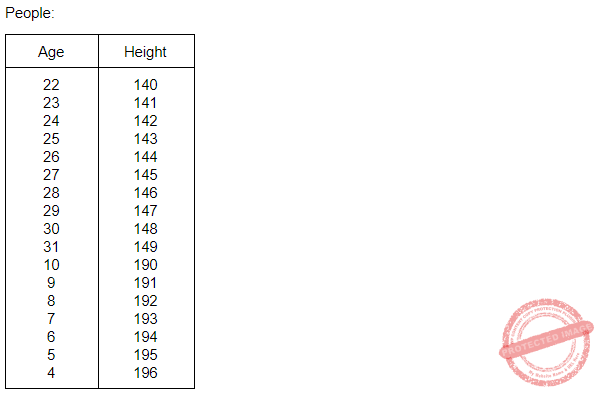→ In this case query (a) will return 10 rows, query (b) will return 7 rows and query (c) will return 17 rows because of “or” condition given in it.
Case II: When result of queries (a) & (b) have the same row.
→ This case result in minimum no. of rows possible for given table.→ In this case maximum 10 rows will be resulted.
Option (C) is most suitable, because 10 is minimum no. of rows possible so option (A) & (B) can’t be correct choice.
And maximum no. of rows possible is 17 so option (D) is also not correct choice.
 Question 62
The distributed system is a collection of (P)and communication is achieved in distributed system by (Q), where (P) and (Q) are:
 A Loosely coupled hardware on tightly coupled software and disk sharing, respectively. B Tightly coupled hardware on loosely coupled software and shared memory, respectively C Tightly coupled software on loosely coupled hardware and message passing, respectively D Loosely coupled software on tightly coupled hardware and file sharing, respectively.
Software-Engineering       Coupling-and-Cohesion
Question 62 Explanation:
A distributed system is a system whose components are located on different networked computers, which communicate and coordinate their actions by passing messages to one another. The components interact with one another in order to achieve a common goal.
Since the components are located on different networked computers so we can say that the hardware are loosely coupled and since they are communicating and coordinating their actions then we can say that software are tightly coupled and the communication is taking place via message passing.
Hence option(C) is the correct answer.
 Question 63
Consider the following three tables R, S and T. In this question, all the join operations are natural joins (⨝). (π) is the projection operation of a relation: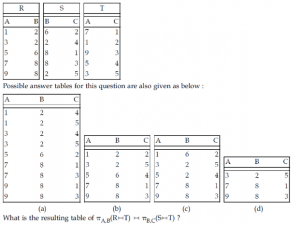A (a) B (b) C (c) D (d)
Database-Management-System       Relational-Algebra
Question 63 Explanation:
Natural join of table R & S results intoQuestion 64
Consider the two class classification task that consists of the following points:
Class C1 : [-1, -1], [-1, 1], [1, -1]
Class C2 : [1, 1]
The decision boundary between the two classes C1 and C2 using single perceptron is given by:
 A x1 - x2 - 0.5 = 0 B - x1 + x2 - 0.5 = 0 C 0.5(x1 + x2) - 1.5 = 0 D x1 + x2 - 0.5 = 0
Artificial-intelligence       Perceptron
Question 64 Explanation:
For such questions,
➜ You should perform multiplication and addition operation between each class matrix and given equations in options.
➜ Option which divides the class into two regions i.e., (+ve & -ve) regions is the correct answer.
Class C1:Question 65
Consider a standard additive model consisting of rules of the form of
If x is Ai AND y is Bi THEN z is Ci.
Given crisp inputs x = x0, y = y0, the output of the model is:
 AB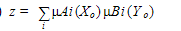C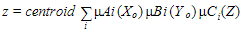DQuestion 66
A bell-shaped membership function is specified by three parameters (a, b, c) as follows:
 A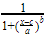BCDArtificial-intelligence       Fuzzy-logic
Question 66 Explanation:Question 67
Which of the following is/are the principal components of a memory-tube display ?
(a)Flooding gun
(b)Collector
(c)Phosphorus grains
(d)Ground
 A (a) and (b) B (c) only C (d) only D All the above
Computer-Graphics       Display-System
Question 67 Explanation:Question 68
Which of the following steps is/are not required for analog to digital conversion?
(a)Sensing
(b)Conversion
(c)Amplification
(d)Conditioning
(e)Quantization
 A (a) and (b) B (c) and (d) C (a), (b) and (e) D None of the above
Data-Communication       A/D-Converter
Question 68 Explanation:Question 69
Which raster locations would be chosen by Bresenham’s algorithm when scan converting a line from (1, 1) to (8, 5)?
 ABCDComputer-Graphics       Bresenham’s-Algorithm
Question 69 Explanation:Question 70
Consider a unit square centred at the origin. The coordinates of the square are translated by a factor (1/2, 1) and rotated by an angle of 90°. What shall be the coordinates of the new square?
 A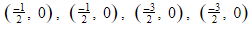BC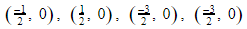DComputer-Graphics       Transformations
Question 70 Explanation: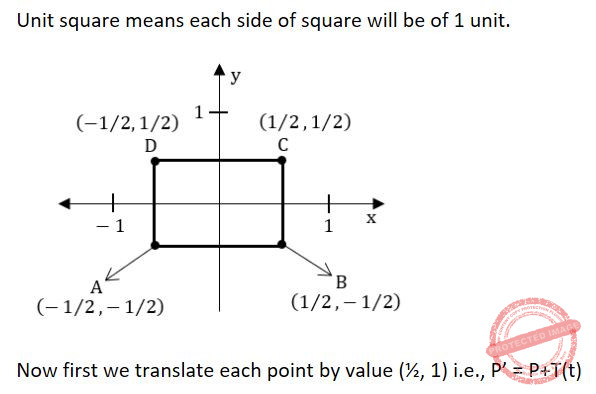Question 71
Which of the following is/are the components of a CRT?
(a)Electron Gun
(b)Control Electrode
(c)Focusing Electrode
(d)Phosphor Coated Screen
 A (a) and (d) B (a), (b) and (d) C (a), (b), (c) and (d) D (a), (c) and (d)
Computer-Graphics       Display-System
Question 71 Explanation: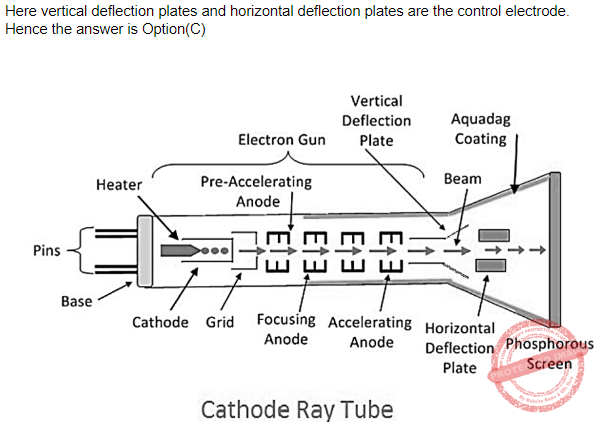Question 72
Which one of the following statements is incorrect?
 A Pareto analysis is a statistical method used for analyzing causes, and is one of the primary tools for quality management. B Reliability of a software specifies the probability of failure-free operation of that software for a given time duration. C The reliability of a system can also be specified as the Mean Time To Failure (MTTF) D In white-box testing, the test cases are decided from the specifications or the requirements
Software-Engineering       Software-testing
Question 72 Explanation:
Option(D) is incorrect because In black box testing, the test cases are decided from the specifications or the requirements.
White box testing is concerned about the Internal functionality of the module are tested that is why it tests the code.
 Question 73
Which one of the following statements, related to the requirements phase in Software Engineering, is incorrect?
 A “Requirement validation” is one of the activities in the requirements phase. B “Prototyping” is one of the methods for requirement analysis. C “Modelling-oriented approach” is one of the methods for specifying the functional specifications D “Function points” is one of the most commonly used size metric for requirements.
Software-Engineering       Software-testing
Question 73 Explanation:
Option(A) is correct because “Requirement verification and validation” is one of the activities in the requirements phase.
Option(B) is correct because we can make a prototype model of a software based upon the customer's basic requirements then the initially developed model can be improved until all customer requirements get fulfilled.
Option(C) is incorrect because “Modelling-oriented approach” is not one of the methods for specifying the functional specifications
Option(D) is correct because “Function points” is one of the most commonly used size metric for requirements.
 Question 74
__________ tag is an extension to HTML that can enclose any number of Javascript statements.
 A < SCRIPT > B < BODY > C < HEAD > D < TITLE >
Web-Technologies       HTML
Question 74 Explanation:Question 75
Consider a language A defined over the alphabet Σ={0, 1} as A={0⌊n/2⌋1n :n>=0}. The expression ⌊n/2⌋ means the floor of n/2, or what you get by rounding n/2 down to the nearest integer.
Which of the following is not an example of a string in A?
 A 011 B 0111 C 0011 D 001111
Theory-of-Computation       Languages-and-Grammars
Question 75 Explanation:
If n=0
A=ε
If n=1
A=1
If n=2
A=011
If n=3
A=0111
If n=4
A=001111
So, A={ε,1,011,0111,001111,...}
Hence, options-(C) is not an example of a string in (A)
There are 75 questions to complete.